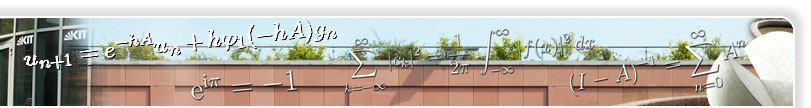# Exponential Integrator for Large Systems of (Stiff) ODEs

```% Exponential Integrator for Large Systems of (Stiff) ODEs.
% ---------------------------------------------------------
%
% This code is an exponential method of order 4 with variable step
% size selection to solve equations of the form y' = f(y), t0 = y(t0).
% A dense output formula to compute the solution at specified
% grid points is also available. The computation of phi(A)*v =
% ((exp(A)-I)/A)*v, where A is the Jacobian matrix of f and v is a
% vector is done by Krylov techniques in the code EXP4 and exactly in
% the code EXP4EX. Therefore EXP4 is the integrator for large systems
% of ordinary differential equations (say, dimension > 100).
%
% For a quick start type 'help bruss2d', 'help laser', 'help heat2d'
% or 'help rob1d' and follow the comments.
%
% The integrators EXP4 and EXP4EX have the same syntax as the MATLAB
% ODE solvers.
%
% For a better description of the codes have a look at:
% Marlis Hochbruck, Christian Lubich, Hubert Selhofer: "Exponential
% Integrators for Large Systems of Differential Equations",
% to appear in SIAM J. Sci. Comp.
%
% The latest versions of the code and the paper are available at
%
%                      https://na.uni-tuebingen.de/
%
% FILES OF THE DISTRIBUTION:
% --------------------------
% The two integrators:
%   * EXP4: The integrator for large problems with Krylov techniques.
%   * EXP4EX: The same integrator as EXP4 without Krylov techniques.
% Utility files of the two integrators:
%   * ERRSTEP: Step size selection based on error estimates.
%   * EXP4_INITIALIZE: Script to initialize the integrators.
%   * H_INIT: Guess an initial step size.
%   * KRYSTEP: Step size selection based on the Krylov process.
%   * KRY_STEP_RED: Helper function for the Krylov processes.
%   * LANCZOS2: Compute the Lanczos approximation.
%   * ODEFUN: Wrapper to evaluate f and df/dy.
%   * PHIM: The function (exp(A)-I)/A for a matrix.
%   * PHI_ARN: The Arnoldi process.
%   * PHI_LAN: The Lanczos process for general A.
%   * PHI_LAN_SYM: The Lanczos process for symmetric A.
%   * PLOTBRUSS2D: Utility to visualize the solution of BRUSS2D.
%   * SC_NORM: Compute a scaled (weighted) norm.
%   * VPRINT: Conditionally print messages.
% Info files:
%   * CHANGE.LOG.EXP4: The changes since the last release.
%   * CONTENTS: This file.
%   * COPYING: Please read this file.
% Examples:
%   * BRUSS2D: Diffusion in 2D.
%   * HEAT2D: Heat equation in 2D.
%   * LASER: Model of an atom-laser-interaction.
%   * ROB1D: Chemical reaction in 1D.
%
% GETTING STARTED:
% ----------------
% The best way is to look at the examples supplied. The easiest
% example is the linear heat equation: HEAT2D. Use this file as a
% prototype for your own problems (see also help odefile). ROB1D and
% BRUSS2D are similar, but introduce a few more features. LASER is the
% most advanced example: It uses an extension to the odefile standard
% where no jacobian matrix has to be explicitly computed. This
% function computes the product of the jacobian matrix times a vector
% using FFTs without ever computing the jacobian itself. This may save
% memory and computation time.
%
% The code EXP4EX is much easier to read and understand than the
% Krylov based EXP4.
% Be brave and follow the help for BRUSS2D and don't forget to look at
% the movie.
%
% ATTENTION:
% This code will NOT run under matlab 4.*.
% Please read the file COPYING of the distribution carefully.
%
% Please send bug reports, experiences, wishes and comments to
% exp4∂na.uni-tuebingen.de.

% This file is part of the exp4 package available at
% https://na.uni-tuebingen.de.
%
% This program is distributed in the hope that it will be useful,
% but WITHOUT ANY WARRANTY; without even the implied warranty of
% MERCHANTABILITY or FITNESS FOR A PARTICULAR PURPOSE.  See the
% file COPYING of the distribution or send email to
% exp4∂na.uni-tuebingen.de.```

Comments and suggestions to www∂am.uni-duesseldorf.de.